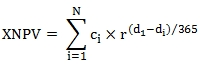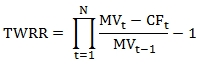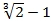## Internal Rate of Return versus Total Return

Apr 23

Written by: Charles Flock
4/23/2015 3:18 PMThere are a lot of financial calculations that compute 'returns'. There are internal rates of return, return on investment, time-weighted rate of return, multiples of invested capital, compound annual growth rate, and many more. The good news is that you can perform all these calculations in SQL Server using XLeratorDB. In this article we explore the difference between the two return calculations that we get the most questions about: internal rate of return and total return. You won't need an MBA in Finance to understand these examples; just follow along with SQL Server Management Studio and you will very quickly see how these calculations differ.
Internal rate of return is a money-weighted return meaning that the amount and the timing of cash movements affect the result. An internal rate of return is calculated using the XLeratorDB XIRR function.
XIRR calculates a discount rate such that the XNPV of the cash flows is approximately zero. Generally, there is no closed-form solution for an internal rate of return and the result is found using iterative techniques.
Given a set of cash flows in ascending date order, the formula for XNPV is:Where

 N = number of cash flows ci = cash flow amount di = date of the cash flow r = 1 + discount arte

Total return, on the other hand, is a time-weighted return; the timing of cash contributions is inconsequential and the calculation captures portfolio or market performance over some time horizon. There are numerous XLeratorDB functions to calculate time-weighted return. For this article, we will use the GTWRR function which uses the following formula.Where

 N = the number of periods MVt = the ending market value for period t MVt-1 = the ending market value for the previous period CFt = the sum of the cash flows greater than the date of MVt-1 and less than or equal to the date of MVt

Let's look at an example.
Given a portfolio of one security, let's assume that the price of the security is flat for years one and two and that the price of the security doubles in year three. Given this information, we will construct two scenarios. In the first scenario there are investments of 10,000 on 2012-12-31 and 10,000 on 2013-12-31. In the second there are investments of 10,000 on 2012-12-31 and 10,000 on 2014-12-31. The value of the position on 2015-12-31 is 40,000. The following SQL calculates the internal rate of return for each scenario.
SELECT
scenario
,wct.XIRR(cf,dt,NULL) as [Internal Rate of Return]
FROM (VALUES
(1,'2012-12-31',-10000)
,(1,'2013-12-31',-10000)
,(1,'2015-12-31',40000)
,(2,'2012-12-31',-10000)
,(2,'2014-12-31',-10000)
,(2,'2015-12-31',40000)
)n(scenario,dt,cf)
GROUP BY
scenario
This produces the following result.
These results tell us that in scenario number 1 the investments earned 31.46% per year compounded annually while in investment number 2 the investments earned 37.88% per year compounded annually. The following SQL demonstrates that.
SELECT
n.scenario
,SUM(-n.cf*POWER(1+x.IRR,wct.YEARFRAC(n.dt,'2015-12-31',3))) as [Ending Value]
FROM (VALUES
(1,'2012-12-31',-10000)
,(1,'2013-12-31',-10000)
,(1,'2015-12-31',40000)
,(2,'2012-12-31',-10000)
,(2,'2014-12-31',-10000)
,(2,'2015-12-31',40000)
)n(scenario,dt,cf)
CROSS APPLY
(VALUES (1,0.314596212276752),(2,0.378796700129481))x(scenario,IRR)
WHERE
n.dt < '2015-12-31'
AND n.scenario = x.scenario
GROUP BY
n.scenario
This produces the following result.
In both scenarios the internal rate of return compounded annually results in the ending value. The differences in the 2 internal rates of return arise solely based on whether or not the second cash flow occurs in 2013 or in 2014. This is why internal rate of return is a money-weighted rate of return,
When calculating a total, or time-weighted, rate of return, we get a much different result.
SELECT
scenario
,wct.GTWRR(amt,dt,mv,1) as [Time-weighted Rate of Return]
FROM (VALUES
(1,'2012-12-31',10000,0)
,(1,'2012-12-31',10000,1)
,(1,'2013-12-31',10000,0)
,(1,'2013-12-31',20000,1)
,(1,'2014-12-31',20000,1)
,(1,'2015-12-31',40000,1)
,(2,'2012-12-31',10000,0)
,(2,'2012-12-31',10000,1)
,(2,'2013-12-31',10000,1)
,(2,'2014-12-31',10000,0)
,(2,'2014-12-31',20000,1)
,(2,'2015-12-31',40000,1)
)n(scenario,dt,amt,mv)
GROUP BY
scenario
This produces the following result.
The time-weighted rate of return is 100% in both cases. In other words, the return was unaffected by the timing of the cash flows.
Notice that the calculation of the time-weighted rate of return included more cash flows than the calculation of the internal rate of return. This is because in addition to the cash flows we also used the end-of-year market-values in the calculation. The following SQL produces a resultant table that splits the cash flows and the market values into separate columns.
SELECT
scenario
,dt
,ISNULL(,0) as CF
, as [Ending Market Value]
FROM (
SELECT
*
FROM (VALUES
(1,'2012-12-31',10000,0)
,(1,'2012-12-31',10000,1)
,(1,'2013-12-31',10000,0)
,(1,'2013-12-31',20000,1)
,(1,'2014-12-31',20000,1)
,(1,'2015-12-31',40000,1)
,(2,'2012-12-31',10000,0)
,(2,'2012-12-31',10000,1)
,(2,'2013-12-31',10000,1)
,(2,'2014-12-31',10000,0)
,(2,'2014-12-31',20000,1)
,(2,'2015-12-31',40000,1)
)n(scenario,dt,amt,mv)
) d
PIVOT(SUM(amt) FOR mv in(,)) pvt
ORDER BY
1,2
This produces the following result.

While it should be obvious, the following SQL shows how the time-weighted rate of return developed over 1, 2, and 3 years in each scenario.
SELECT
scenario
,x.dt
,x.trm as [term]
,wct.GTWRR(n.amt,n.dt,n.mv,1) as [Time-weighted Rate of Return]
FROM (VALUES
(1,'2012-12-31',10000,0)
,(1,'2012-12-31',10000,1)
,(1,'2013-12-31',10000,0)
,(1,'2013-12-31',20000,1)
,(1,'2014-12-31',20000,1)
,(1,'2015-12-31',40000,1)
,(2,'2012-12-31',10000,0)
,(2,'2012-12-31',10000,1)
,(2,'2013-12-31',10000,1)
,(2,'2014-12-31',10000,0)
,(2,'2014-12-31',20000,1)
,(2,'2015-12-31',40000,1)
)n(scenario,dt,amt,mv)
CROSS APPLY(VALUES
('1Y','2013-12-31'),('2Y','2014-12-31'),('3Y','2015-12-31')
)x(trm,dt)
WHERE
n.dt <= x.dt
GROUP BY
n.scenario
,x.dt
,x.trm
ORDER BY
1,2
This produces the following result.
It's tempting to turn the total return into an annual return and that is relatively easy to do in this case. A 100% return over 3 years == 0.25991. However, it is impossible to take that value and apply it to the cash flows and come up with the ending values. In fact, what happened is that the investment was flat for 2 years and then doubled in the third year.
Which calculation is right for you? Obviously that depends on what it is you are trying to measure. If you want a rate that you can apply to a series of cash flows that will get you to an ending balance, then internal rate of return is the calculation that you want. If you want to measure how an investment or an investment manager performed over some time horizon and you want remove the impact of cash movements, then time-weighted rate of return is the calculation that you want.

You can try out these calculations for yourself by downloading the free 15-day trial of XLeratorDB right now and installing it on you SQL Server. Then just copy the examples into SMS and run them. XLeratorDB has over 900 functions for in-database analytics that can turn your SQL Server into an analytics engine.

Tags:
Categories:
Location: BlogsThe WestClinTech Blog

## Search Blogs

 KeywordsPhrase

## Blog Archives

Archive
 < September 2023 >
SunMonTueWedThuFriSat
272829303112
3456789
10111213141516
17181920212223
24252627282930
1234567
Monthly
Go

### SupportCopyright 2008-2023 Westclintech LLC         Privacy Policy        Terms of Service